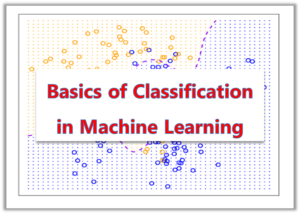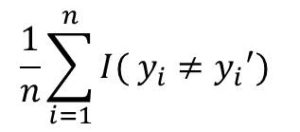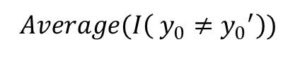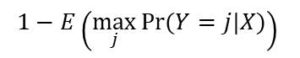# Classification in Machine Learning (A Simple Tutorial for Beginners)

In this article, we would explain the concept of classification in a very clear and easy to understand manner.We would cover the following:

#### 1.  Introduction to Classification

Assuming we are given a dataset  { (x1,y1), (x2,y2),…,(xn,yn)}

This dataset is the training dataset. This is such that each of the x values belongs to a class y. That is x1 belongs to class y1, x2 belongs to class y2 and so on.

The goal is to create a model that is trained with the training dataset such that when the model encounters a new value of x which is does not have a class, it would be able to classify it correctly.  (Variables y are described as qualitative. This means that they are not continuous. Examples is 1 and 0)

The question now is: how do we classify x correctly and how do we check for the accuracy of our classification?

#### 2. Training and Test Error Rate

The error rate explains the percentage of the classification that is done wrongly. That is number of wrong classification divided by total number of observations. So if there are 100 observations, x1 to x100 (n is 100) and we classify 20 correctly, then the error rate would be 20/100 = 0.2.

In other words, if the observation xi belongs to class yi and we classify it as yi, then we can sum up all the occurrences where we classified yi as yi. This is done using an indicator random variable I as shown below.In the expression, yi is the class predicted for xi where xi is the ith observation.

I(yi ≠ yi’) is an indicator random variable which is equal to  1 if the classification is wrong, that is  yi ≠ yi.

And I equals 0 if yi = yi

So basically, we are counting the number observations that were misclassified, and that give us the error rate. if we divide by the total number of observations n.

This is what is known as the training error rate since we are calculating the error in classifying the training data set. Then we also have the test error rate if the data set is the test data set. For a set of test data (x0, y0), the test error rate is given byThe goal of Classification is the minimize the test error rate.

#### 3. The Bayes Classifier

Bayes Classifier is a types of classifier that performs classification by applying Bayes Theorem. They are also know as probabilistic classifiers.

The next logical question is ‘What is Bayes Theorem?’

Bayes theorem, also known as Bayes rule is a theorem in statistics used to determine the probability of an unknown event from another known event that is related to the unknown one.

In Bayes classifier, we assign the observation to the class with the highest probability. This can be stated this way:

Pr(Y = j | X = x0)

This simply means the probability that Y = j given the observation x0.

Let’s assume that there are only two classes, class 1 and class 2. You can think of this as a binary classifier where there are two classes 1 and 0. In this case, Bayes classifier would predict for class 1 if Pr(Y = j | X = x0) > 0.5 and class 2 if Pr(Y = j | X = x0) < 0.5

#### 4. Bayes Decision Boundary

When Bayes classifier is used, it creates a decision boundary between observation of the two classes. For on class, the conditional probability greater than 0.5, and for the other class, the conditional probability is less than 0.5.  But there are points where the conditional probability is exactly 0.5. This forms the Bayes Decision Boundary.

This is shown in Figure 1.2.

#### 5. Bayes Error Rate

Just as you can guess, the Bayes Error Rate is a the error rate associated with Bayes Classifier and is described as the lowest possible error rate for probabilistic classifiers.

The Bayes Error Rate is given by the formula:Here is is the Expectation and is the average of the probabilities over all the possible value of X.#### kindsonthegenius

Kindson Munonye is currently completing his doctoral program in Software Engineering in Budapest University of Technology and Economics

View all posts by kindsonthegenius →